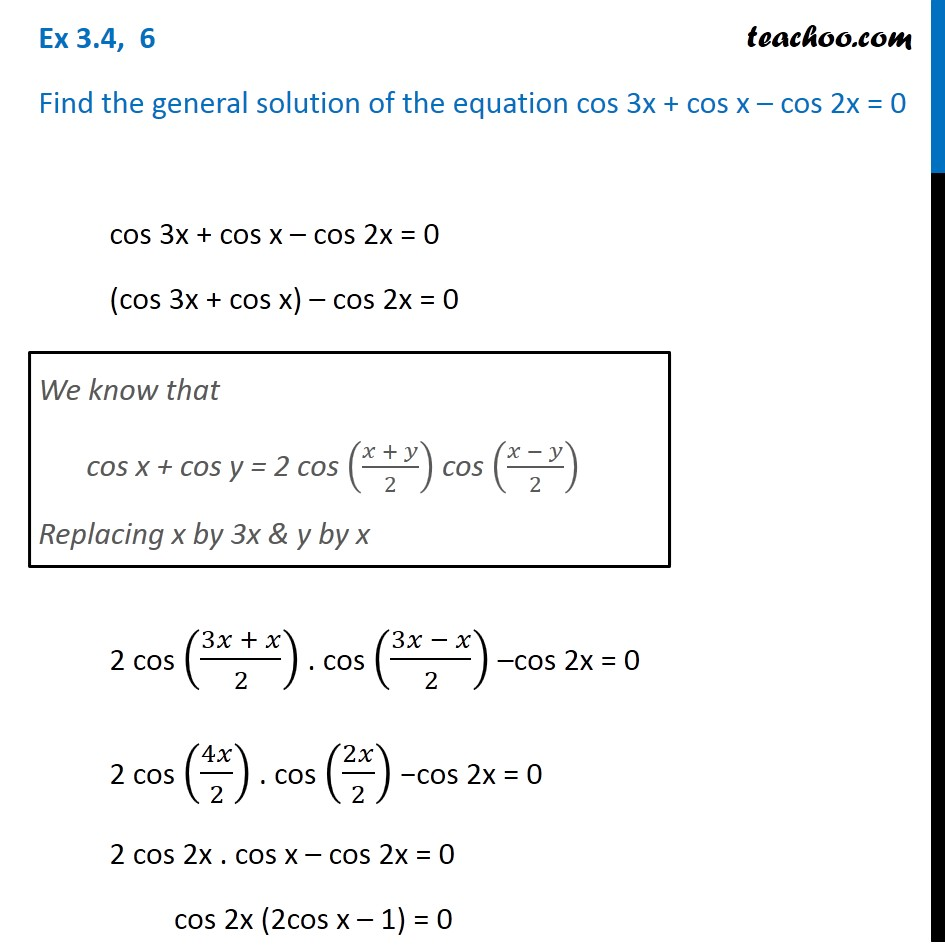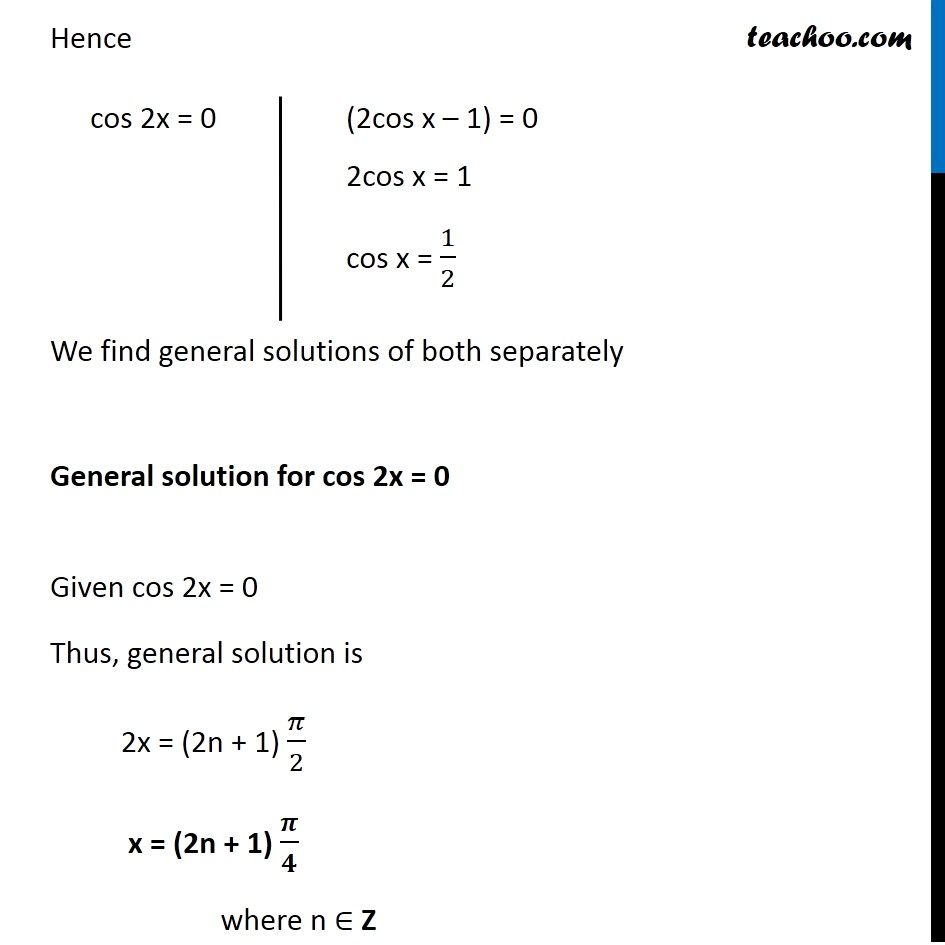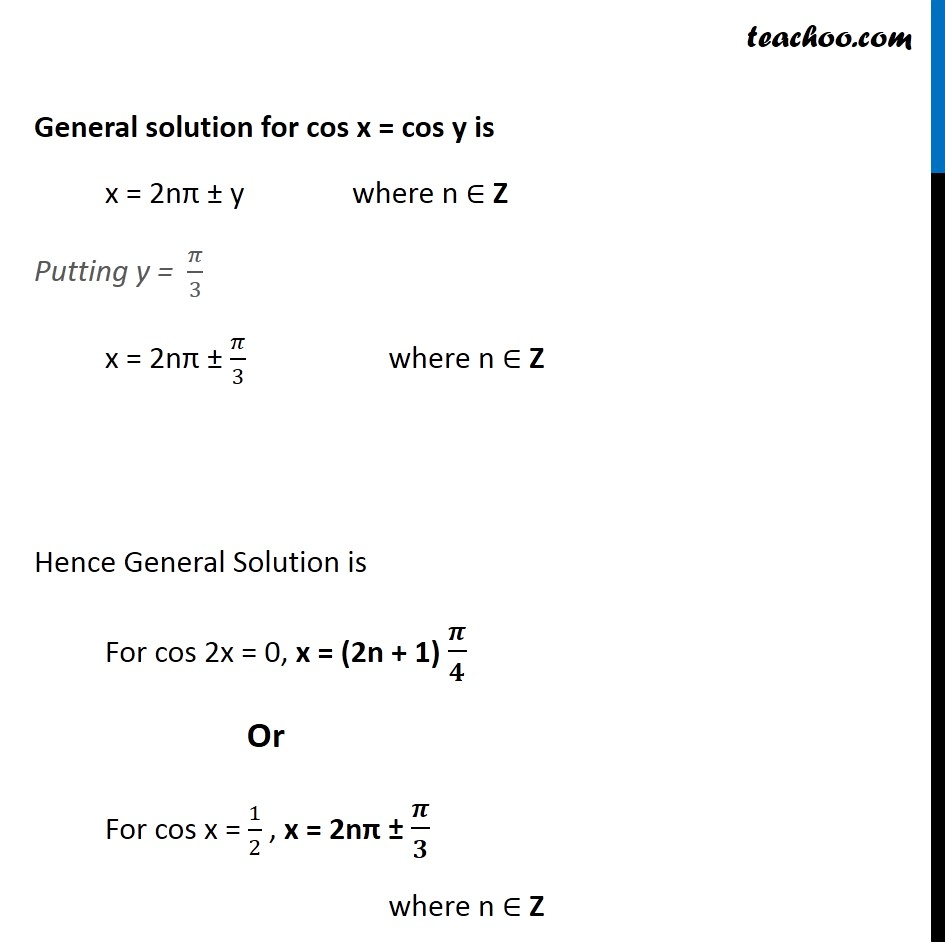Finding General Solutions

Chapter 3 Class 11 Trigonometric Functions
Concept wiseIntroducing your new favourite teacher - Teachoo Black, at only ₹83 per month

### Transcript

Ex 3.4, 6 Find the general solution of the equation cos 3x + cos x – cos 2x = 0 cos 3x + cos x – cos 2x = 0 (cos 3x + cos x) – cos 2x = 0 2 cos ((3𝑥 + 𝑥)/2) . cos ((3𝑥 − 𝑥)/2) –cos 2x = 0 2 cos (4𝑥/2) . cos (2𝑥/2) −cos 2x = 0 2 cos 2x . cos x – cos 2x = 0 cos 2x (2cos x – 1) = 0 We know that cos x + cos y = 2 cos ((𝑥 + 𝑦)/2) cos ((𝑥 − 𝑦)/2) Replacing x by 3x & y by x Hence We find general solutions of both separately General solution for cos 2x = 0 Given cos 2x = 0 Thus, general solution is 2x = (2n + 1) 𝜋/2 x = (2n + 1) 𝝅/𝟒 where n ∈ Z (2cos x – 1) = 0 2cos x = 1 cos x = 1/2 General solution for cos x = 𝟏/𝟐 Let cos x = cos y Given cos x = 1/2 From (3) and (4) cos y = 1/2 cos y = cos (𝜋/3) y = 𝜋/3 Rough We know that cos 60° = 1/2 So, 60° = 60 × 𝜋/180 = 𝜋/3 General solution for cos x = cos y is x = 2nπ ± y where n ∈ Z Putting y = 𝜋/3 x = 2nπ ± 𝜋/3 where n ∈ Z Hence General Solution is For cos 2x = 0, x = (2n + 1) 𝝅/𝟒 Or For cos x = 1/2 , x = 2nπ ± 𝝅/𝟑 where n ∈ Z